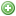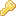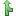FAQSearchRegisterLogin

## Set16ChannelRaw Unexpected behavior

Related to the development libraries, released by Curt BinderjoshlawlessPosts: 138Joined: Thu May 23, 2013 2:52 pmPosted: Wed Aug 26, 2015 9:12 pm It looks like it should beCode: Select all`else if ((end - current) < slopeLengthSecs){ // it's in the end slope downsmoothPhase = (((float)(end-current)/(float)slopeLengthSecs)*180.0) + 180.0; `This way, smoothPhase varies from 2pi to pi over the final slope, the cosine of smoothPhase varies from 1 to -1 over the final slope,half the sum of smoothPhase and 1.0 varies from 1 to 0 over the final slope,and the return value varies from endPWMint to startPWMint over the final slope Last edited by joshlawless on Fri Aug 28, 2015 3:19 pm, edited 1 time in total.TopjoshlawlessPosts: 138Joined: Thu May 23, 2013 2:52 pmPosted: Fri Aug 28, 2015 12:57 pm Just a follow-up to state that after making the change specified in the post above to my copy of the libraries, I can confirm the behavior is now as I would expect.ChartOne thing I noticed when parsing the function -- it calculates the value based on the current time in seconds. With a 12-bit slope, though, you would need at least a 70 minute (linear) ramp to take full advantage of all 4095 levels available. I wonder if it isn't possible to increase the resolution to tenths (or even hundredths) of a second, e.g., by multiplying the start, end and current values by 10, and adding (current_millis()%1000 - current_millis()%100)/100 to the current value, as in this function (which also takes PWM start and end values as 12-bit values instead of percentages):Code: Select all`int PWMSmoothRampHighestRes(byte startHour, byte startMinute, byte endHour, byte endMinute, int startPWMint, int endPWMint, byte slopeLength, int oldValue){  LightsOverride=true;  int current_hour = hour();  long current_millis = millis()  long start = NumMins(startHour, startMinute)*600L;  long end = NumMins(endHour, endMinute)*600L;  long slopeLengthTenthSecs = slopeLength*600L;  if (start > end) // Start is greater than End so it is over midnight  {    if (current_hour < endHour) start -= 1440L*600L; // past midnight    if (current_hour >= startHour) end += 1440L*600L; // before midnight  }  long current = NumMins(current_hour, minute())*600L + second()*10L + (current_millis()%1000 - current_millis()%100)/100L;  if (slopeLengthTenthSecs > ((end-start)/2) ) slopeLengthTenthSecs = (end-start)/2; // don't allow a slope length greater than half the total period  if (current <= start || current >= end)     return oldValue; // it's before the start or after the end, return the default  else  { // do the slope calculation    int pwmDelta = endPWMint - startPWMint;    float smoothPhase;    if ((current > (start + slopeLengthTenthSecs)) && (current < (end - slopeLengthTenthSecs)))       return endPWMint; // if it's in the middle of the slope, return the high level    else if ((current - start) < slopeLengthTenthSecs)     {  // it's in the beginning slope up      smoothPhase = (((float)(current-start)/(float)slopeLengthTenthSecs)*180.0) + 180.0;    }    else if ((end - current) < slopeLengthTenthSecs)    { // it's in the end slope down      smoothPhase = (((float)(end-current)/(float)slopeLengthTenthSecs)*180.0) + 180.0;    }    return startPWMint + (int)(pwmDelta*((1.0+(cos(radians(smoothPhase))))/2.0));  }}`ToplnevoPosts: 5402Joined: Fri Jul 20, 2012 9:42 amPosted: Sat Aug 29, 2015 4:16 am Looks awesome. I'm not sure how much sense it would make to change it to tenths but I'm intrigued by your approach. I've been thinking of that for a bit with my dosing pump code. Because I'm dosing by volume at the end of the day I'm dosing a little bit under maybe by a full ml because of the resolution there. Interested in helping to take a stab there?Lee's Feature Complete PDETopjoshlawlessPosts: 138Joined: Thu May 23, 2013 2:52 pmPosted: Sat Aug 29, 2015 11:11 am lnevo wrote:Looks awesome. I'm not sure how much sense it would make to change it to tenths but I'm intrigued by your approach. I've been thinking of that for a bit with my dosing pump code. Because I'm dosing by volume at the end of the day I'm dosing a little bit under maybe by a full ml because of the resolution there. Interested in helping to take a stab there?Sure thing. For posterity, here's an updated/corrected version of the function above -- the one above had some typos (missing ";" and accidentally included "()" after current_mills. Code: Select all`int PWMSmoothRampHighestRes(byte startHour, byte startMinute, byte endHour, byte endMinute, int startPWMint, int endPWMint, byte slopeLength, int oldValue){  LightsOverride=true;  int current_hour = hour();  long current_millis = millis();  long start = NumMins(startHour, startMinute)*600L;  long end = NumMins(endHour, endMinute)*600L;  long slopeLengthTenthSecs = slopeLength*600L;  if (start > end) // Start is greater than End so it is over midnight  {    if (current_hour < endHour) start -= 1440L*600L; // past midnight    if (current_hour >= startHour) end += 1440L*600L; // before midnight  }  long current = NumMins(current_hour, minute())*600L + second()*10L + (current_millis%1000 - current_millis%100)/100L;  if (slopeLengthTenthSecs > ((end-start)/2) ) slopeLengthTenthSecs = (end-start)/2; // don't allow a slope length greater than half the total period  if (current <= start || current >= end)     return oldValue; // it's before the start or after the end, return the default  else  { // do the slope calculation    int pwmDelta = endPWMint - startPWMint;    float smoothPhase;    if ((current > (start + slopeLengthTenthSecs)) && (current < (end - slopeLengthTenthSecs)))       return endPWMint; // if it's in the middle of the slope, return the high level    else if ((current - start) < slopeLengthTenthSecs)     {  // it's in the beginning slope up      smoothPhase = (((float)(current-start)/(float)slopeLengthTenthSecs)*180.0) + 180.0;    }    else if ((end - current) < slopeLengthTenthSecs)    { // it's in the end slope down      smoothPhase = (((float)(end-current)/(float)slopeLengthTenthSecs)*180.0) + 180.0;    }    return startPWMint + (int)(pwmDelta*((1.0+(cos(radians(smoothPhase))))/2.0));  }}`Top
Previous